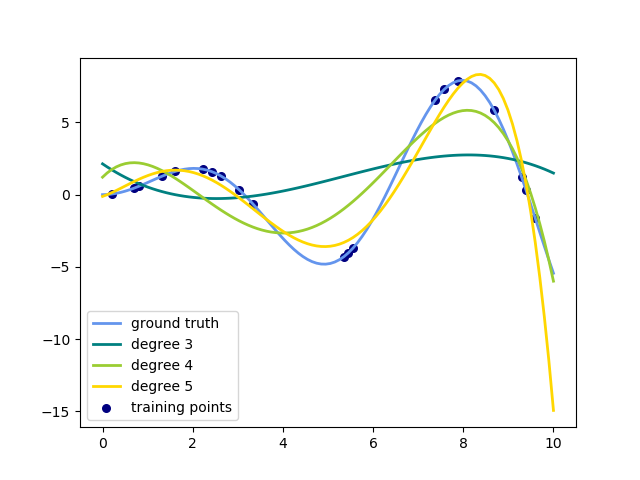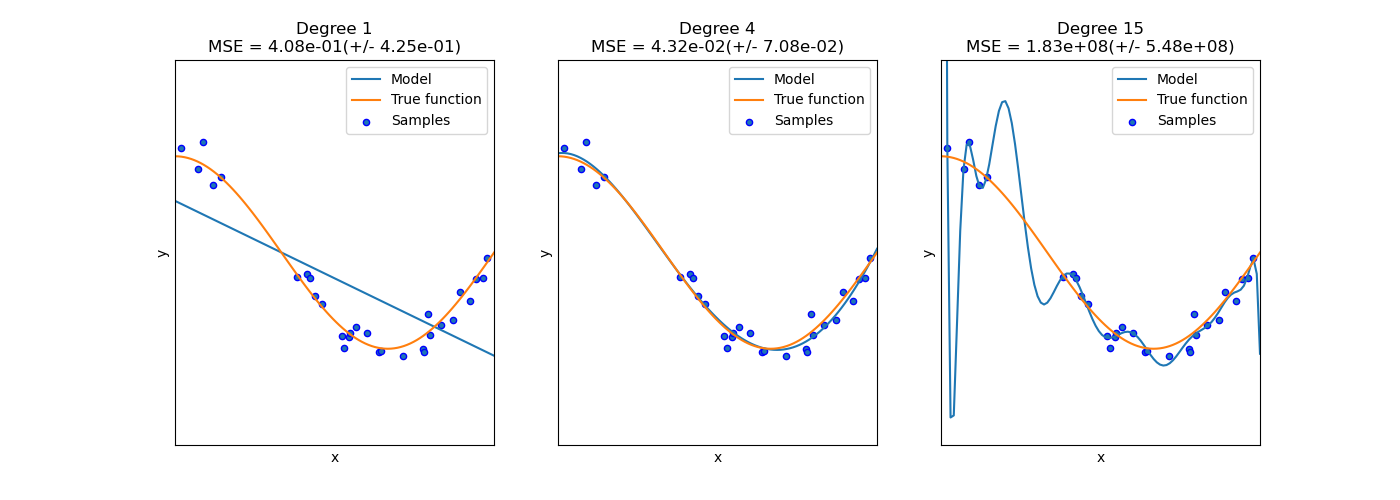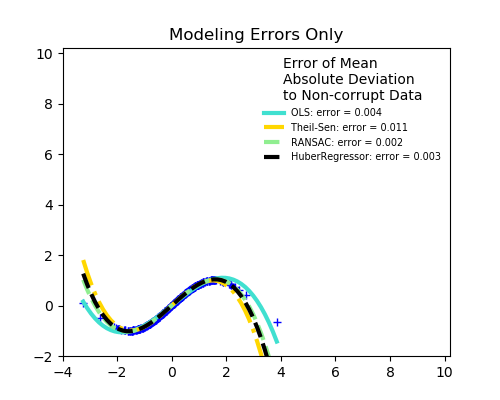# sklearn.preprocessing.PolynomialFeatures¶

class sklearn.preprocessing.PolynomialFeatures(degree=2, *, interaction_only=False, include_bias=True, order='C')

[源码]

degree integer

interaction_only boolean, default = False

include_bias boolean

order str in {‘C’, ‘F’}, default ‘C’

0.21版中的新功能。

powers_ array, shape (n_output_features, n_input_features)
powers_ [i，j]是第i个输出中第j个输入的指数。
n_input_features_ int

n_output_features_ int

>>> import numpy as np>>> from sklearn.preprocessing import PolynomialFeatures>>> X = np.arange(6).reshape(3, 2)>>> Xarray([[0, 1],       [2, 3],       [4, 5]])>>> poly = PolynomialFeatures(2)>>> poly.fit_transform(X)array([[ 1.,  0.,  1.,  0.,  0.,  1.],       [ 1.,  2.,  3.,  4.,  6.,  9.],       [ 1.,  4.,  5., 16., 20., 25.]])>>> poly = PolynomialFeatures(interaction_only=True)>>> poly.fit_transform(X)array([[ 1.,  0.,  1.,  0.],       [ 1.,  2.,  3.,  6.],       [ 1.,  4.,  5., 20.]])

fit(X[, y]) 计算输出要素的数量。
fit_transform(X[, y]) 拟合数据，然后对其进行转换。
get_feature_names([input_features]) 返回输出要素的要素名称
get_params([deep]) 获取此估计量的参数。
set_params(**params) 设置此估算器的参数。
transform(X) 将数据转换为多项式特征
__init__(degree=2, *, interaction_only=False, include_bias=True, order='C')

[源码]

fit(X, y=None)

[源码]

X array-like, shape (n_samples, n_features)

self instance
fit_transform(X, y=None, **fit_params)

[源码]

X {array-like, sparse matrix, dataframe} of shape (n_samples, n_features)
y ndarray of shape (n_samples,), default=None

**fit_params dict

X_new ndarray array of shape (n_samples, n_features_new)

get_feature_names(input_features=None)

[源码]

input_features list of string, length n_features, optional

output_feature_names list of string, length n_output_features
get_params(deep=True)

[源码]

**params dict

self object

transform(X)

[源码]

X array-like or CSR/CSC sparse matrix, shape [n_samples, n_features]

XP np.ndarray or CSR/CSC sparse matrix, shape [n_samples, NP]

## sklearn.preprocessing.PolynomialFeatures使用示例¶